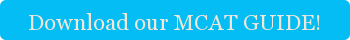This is the second post in a series of indeterminate length and frequency on the best MCAT test tips for the physical sciences section.

MCAT test preparation is a lot about efficiency. The best MCAT preparation involves knowing the most common MCAT topics backwards and forwards. And if there’s one topic MCAT physics loves to ask about, it’s free fall. Free fall problems are a great place to focus your attention as you’re studying for the MCAT for a couple of reasons. First, they’re a great way to practice the kinematics knowledge that you need for other areas of the test. Second, there is a set of shortcuts that you can practice to help you breeze through these problems and spend more time on other parts of the physical sciences section.

#### First, let’s review some concepts.

All objects on earth are accelerated in a downwards direction. This acceleration is 9.81 m/s2, though for the MCAT, we round it to 10 m/s2. In fact, when you’re studying for the MCAT, you should only use 10, never 9.81; it’s always good enough. For the purposes of this article, free fall is any situation where an object starts at rest and then falls, with only gravity acting on it.

You don’t need an MCAT tutor to tell you that the distance an object travels can be summed up by this formula: d=1/2*a*t2  (we’re assuming the object is starting from rest, so vo=0). But a great MCAT tutor (like the ones you find at Cambridge Coaching) will tell you that for free fall, you can simplify this formula to d=5t2! All we did was sub in g (10 m/s2)*1/2. So suppose you have to figure out how long your MCAT books take to fall after you push them out a window 80 m above the street? Well you just set 80=5t2 and solve for t! If you got 4 seconds, you did it right. This is the best MCAT preparation; specific strategies that will save you time on specific problems.

The real power of free fall strategies are realizing just how many situations it applies to. For example, imagine your MCAT tutor threw a rock straight up in the air, at 100 m/s (she has a really strong arm). Is that free fall? Not at first, but at some point the rock will reach an apex in the air, stop, and fall back down. Since the back half of the motion is free fall, we know that the front half of the motion will be exactly the same in reverse.

#### So first question is, how long will the rock be in the air?

Well, since we started at v=100 m/s, and g=-10 m/s2 (here g is negative because it is in the opposite direction as the initial velocity), we can divide to get that t=10 seconds. And since we know that the second half of the motion is the same as the first in reverse, we know that the rock stays in the air for 20 seconds! Another MCAT preparation tip-always do problems in the shortest way possible; time is not on your side.

Now that we know the rock travelled up for 10 seconds, how high did it get? Since we’ve been studying for the MCAT using our best MCAT preparation techniques, we know we can use the free fall formula above (d=5t2). Did you get 500 m? If so you got the right answer. And you got it quickly and efficiently (that’s the point of these MCAT test tips!).

Not everyone needs an MCAT tutor, but just because you’re getting all the answers doesn’t mean you’re using the best MCAT preparation strategies. The MCAT is about quick decisions and using your time wisely.  An MCAT tutor can help teach you short cuts that will let you blaze through the easy problems and spend more time on the tough ones.Tags: MCAT